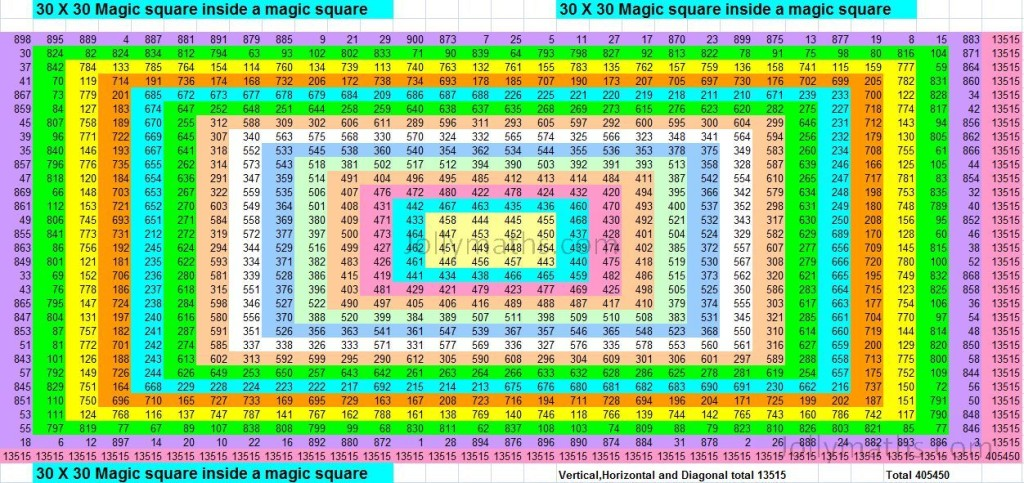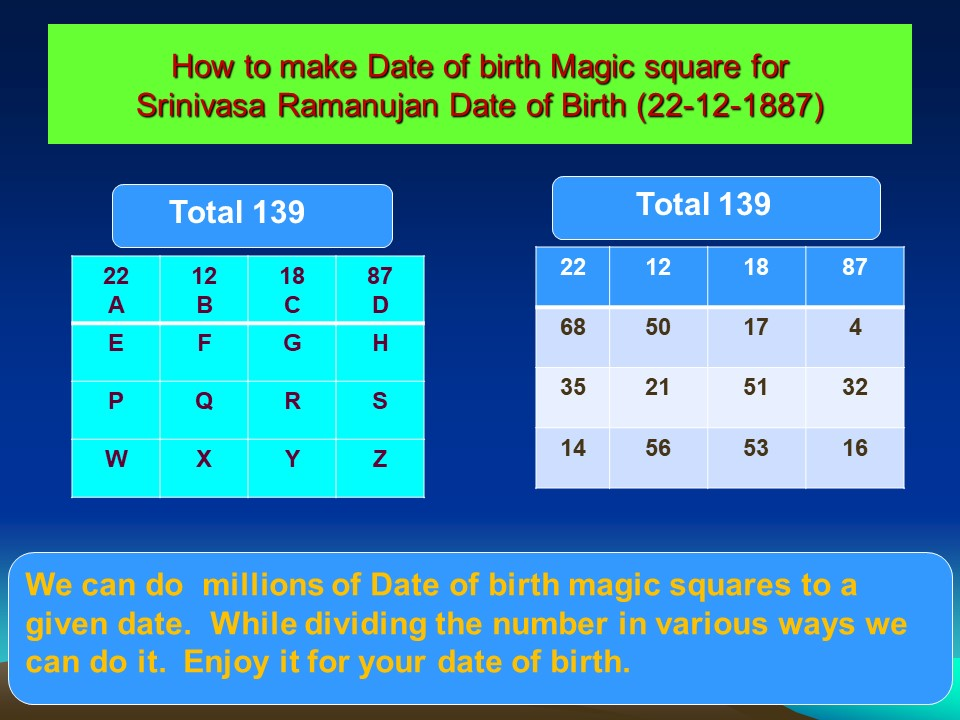# 9×54 Ramanujan sudoku

This is a variation of the popular puzzle Sudoku.

The word “RAMANUJAN” occupied 89 cells and numbers 1 to 9 are used 9 times each and the balance filled with 1 to 8. 8 along with the properties of NORMAL SUDOKU.

1) The entire word “RAMANUJAN” is first written using 119 squares (or cells).
2) Numbers 1 to 9 are filled inside each of these letters.
3) In total, the numbers 1 to 9 are written 13 times and the remaining cells are filled with 1 and 2.
4) We then take each Sudoku of 9 x 9 individually and fill them. The total number of squares that are covered for the word/letter are filled 1 to 9, the adjacent Sudoku (in the right) will commence with the numbers next to the last with 1 to 9 till it fills in the alphabet in that 9 x 9 Sudoku, and the next word/letter will start with the filled number.
For example, the first sudoku contains 20 squares, that are filled with 1 to 9 two times and the balance with 1 & 2. Hence the next Sudoku will commence with 3 to 9, 1 to 9, and so on.

# DIAMOND inlaid Magic Square

This is a DIAMOND Magic-Square wherein all the ODD-numbers are inside the Diamond shape. (Magic-Total is 65)

[table id=diamond_inlaid_5x5 /]

# 30 x 30 Magic Squares inside a Magic SquareThe above is a 30 x 30 Magic Squares inside a Magic Square

This is a special construction of a Magic Square wherein we start with a small Magic Square (centermost one in the figure) and added rows and columns around it, ensuring that we have a Magic Square at each stage. This Magic Square has been color-coded to make the Magic Squares clearer.

From the center Yellow coloured is a 4 x 4 Magic square with a total of 1802 in all vertical, Horizontal and in both diagonals. When combininng with the blue coloured sqauare,  that 6 x 6  will give a totl of 2703.  Further it will expand to 8 x 8 , 10 x 10 etc… and finally it will become 30 x 30 with a grand total of 13515.

I have done this Magic squares inside the magic square from 5 x 5,     6 x 6, 7 x 7, …….198 x 198, 199 x 199 and 200 x 200.  In the 200 x 200 Magic squares inside a magic square all the numbers from 1 to 40,000 are used and the row total will be 4,00,00,100.  Also I am  planning to do it upto 1500 x 1500.

Definitely, it is a gift given to me by Almighty.  I thank the Almighty for giving me the idea, guided me to work, and helped me to complete it with much difficulties.

# SWASTIKA Magic Square

This is a special Magic Square called as the SWASTIKA Magic Square. All odd numbers are inside the SWASTIKA SYMBOL. (The Magic-Total is 65)

[table id=swastika-5×5 /]

You can find more special Magic-Squares here

# How to construct a Magic Square for a Given Date

Let us do magic square to the date of Birth of our Greatest  Indian Mathematician Sri Srinivasa Ramanujan.

His date of Birth is 22nd Dec 1887. The following is a Date-Of-Birth Magic Square with a Magic Total 139.

By following the given method we can do hundreds of, thousands of, millions of Date of Birth Magic Squares for any given date.

The following is a table with variables, which we will use in the explanation

#####1. Write the date in the top row first square, month in the second square and year in two parts in the third and fourth square.
2. Add all the four numbers and write on the top of the square (139).
3. Now we have to make a 4 x 4 magic square.
4. Draw a empty 4 x 4 square and replace 22 by A,   12 by B,   18 by C and 87 by D.
5. Now we know  A,B,C and D.   Rest of the values we have to find out.
6. Count all numbers in the total 1+3+9=13. Once again count  1+3=4. (add all numbers and make it a single digit. Write this in the H square
7. Now add B+C ( 12 + 18 =30). Divide 30 into two parts. i.e 14 and 16. Write 14 in W square and 16 in the Z square
8. By using the properties of Magic square, i.e all vertical, Horizontal and both Diagonal totals are equal, we are going to solve this Magic Square.
9. In the Fourth vertical column, we know the values of D,H,Z. we have to find Out the value of S. Hence S = 139 – (D+H+Z) = 139 — ( 87+4+16) = 139 – 107 = 32. This is the value of S. Write 32 in the S square
10. Now in Diagonals, we know the value of A and Z . Hence the value of F + R = 139 – (22 + 16) = 139-38= 101. Divide it into two parts 50 and 51 and write it in the F and R squares. F = 50 and R = 51.
11. In another diagonal, we know the value of D and W . Hence the value of  G+Q = 139 – (87 + 14) = 139-101= 38. Divide it into two parts 17 and 21 and write it in the F and R squares. G = 17 and Q = 21.
12. In the second row, we know the value of E,G,H. Hence value of E=139-(E+G+H)= 139 –( 50+17+4)= 139 – 71 = 68. Write 68 in E.
13. In the third row, we know the value of Q,R,S. Hence value of P=139-(Q+R+S)= 139 –(21+51+32)= 139 – 104 = 35. Write 35 in P.
14. Now in the second vertical column, we know the values of B,F,Q. Hence value of X = 139 – (12+50+21) = 139- 83 = 56. Write 56 in X
15. Now in the third vertical column, we know the values of C,G,R. Hence value of X = 139 – (18+17+51) = 139- 86 = 53. Write 53 in Y
16. Now add the values of W,X,Y and Z. You will  get 139 is the total.

Hurray!!! MAGIC SQUARE FOR 22-12-1887 IS READY

In step number 10, we have divided 101 into 50 and 51.  Instead it can be divided into q and 101 or 1 and 100 or 2 and 99 etc. etc… Interestingly we can divide it as -1 and 102 or -2 and 103 or -3 ans 104 etc.  While dividing each number, if the numbers are slightly changed, we can get a new Magic square.

Hence we can make hundreds of, thousands of, millions of date of birth magic squares for any given date

Enjoy Magic square for your date of Birth!!!    In various methods….

# How to construct a 3×3 Magic Square for a Given Total

Let the given Number be 63. We will get to the following 3 X 3 Magic-Total 63.

[table id=construct-magic-square-for-given-total-63__3x3 /]

1. Let us do 3 x 3 magic square with Magic Sum of 63.
2. From 63, minus 15 ( the row total of 3×3 base magic square. (63-15=48)
3. Divide 48 by 3(since we have decided to do 3×3 magic square (48/3=16).
4. Add one to this (16+1=17)
5. Now start constructing a 3 x 3 magic square with 17 as the beginning number and (Top row middle – Just in the place of 1).

Note:

• For constructing 3×3 magic square -15, then divide by 3, then add 1.
• For constructing 4×4 magic square -34, then divide by 4, then add 1. and
• For constructing 5×5 magic square -65, then divide by 5, then add 1.

While dividing by 3 or 4 or 5, if any fraction comes, put that fraction in all the squares. You should not delete or make it decimal.

# How to construct 6 x 6 Magic square

Here is a 6 x 6 Magic Square with Magic-Total 111

1)Draw a 6 x 6 empty square.

2)Draw a bold line after the third square, Horizontally and vertically.

3).Now the 6 x 6 magic square will be divided into four 3 x 3 Magic                         squares.

4) Start filling the 3 x 3 magic square on the top left with numbers 1 to 9. and top right from 19 to 27, bottom left with 28 to 36 and bottom right with 10 to 18.

5) Now exchange the numbers 8,5,4 from the top left 3 x 3 square to the bottommost left  3 x 3 squares with the numbers 35, 32, 31 and vice versa.

6) Your 6 x 6 magic square is ready. All vertical, Horizontal and both diagonal totals are equal ( 111) .

As per the instructions given in step no. 4, the numbers 35,32,31 will be available in the down magic square in place of 8,5,4

The FINAL FORMAT OF 6 X 6 IS GIVEN ABOVE. This will be after exchanging numbers 8,5,4 and replacee them by 35,32,31 and vice versa

# How to construct a 4 x 4 magic square

This is a 4 x 4 Magic Square with Magic-Total 34

[table id=4×4-magic-square /]

1. Draw a 4×4 empty square.
2. Draw two diagonals.
3. Start counting all the squares from the top left towards right.
4. Now start writing numbers 1 to 16, count all squares and write numbers ONLY IN THE BLANK SQUARES (2,3,5,8,9,12,14,15 are to be written)
5. Now start writing number from the bottom most right square towards left, count all squares, BUT WRITE NUMBERS ONLY IN THE SQUARES HAVING DIAGONAL LINES ONLY (1,4,6,7,10,11,13 AND 16) ARE TO BE WRITTEN.
6. Magic square 4×4 is ready.

Note:  This method is applicable only for 4×4 magic square.  For 6×6, 8×8 there are separate methods available.

# How to construct an odd order magic square?

This is a 3 X 3 Magic square with Magic-Total 15

[table id=3×3-magic-square__total-15 /]

We will discuss the construction of this, step-by-step.

1.  Put number one in the upper middle square.
2. Always write the next number ONE SQUARE RIGHT AND ONE SQUARE ABOVE
3. AND WHEN IT GOES:
• Beyond boundary in the upper side, write it vertically down most square.
• Beyond the boundary in the right side, write it in the horizontally leftmost Square.
• When the square is already filled with a number, just step-down one square vertically below.
4. And after the Top right square always write the next number one square vertically below.

With these simple steps you can make any biggest “ODD NUMBER MAGIC SQUARE IN THE WORLD”

These steps are useful ONLY FOR ODD NUMBER MAGIC SQUARE AND WILL NOT BE USEFUL FOR EVEN NUMBER MAGIC SQUARES.